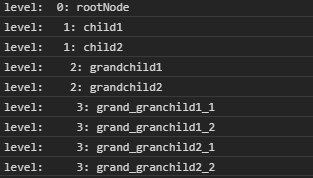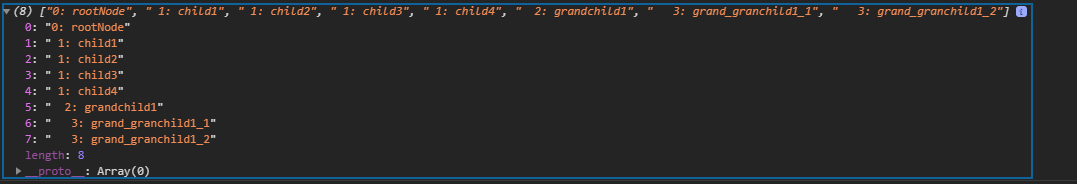# D3.js node.each() Function

• Last Updated : 31 Aug, 2020

The node.each() function is used to evaluate a function for each node in Breadth First Order. In this, every node is visited exactly one time. This function is called repeatedly for each descendant node.

Syntax:

Hey geek! The constant emerging technologies in the world of web development always keeps the excitement for this subject through the roof. But before you tackle the big projects, we suggest you start by learning the basics. Kickstart your web development journey by learning JS concepts with our JavaScript Course. Now at it's lowest price ever!

`node.each(function);`

Parameters: This function accepts a single parameter as mentioned above and described below:

• function: This takes a function to be called on each node in the BFS order.

Return Values: This function does not return anything.

Example 1:

## HTML

 ` ``<``html` `lang` `= ``"en"``> `` ` `<``head``> ``    ``<``meta` `charset` `= ``"UTF-8"` `/> ``    ``<``meta` `name` `= ``"viewport"``          ``path1tent = "``width``=``device``-width, ``          ``initial-scale` `= ``1``.0"/> `` ` `    ``<``script` `src` `=``        ``"https://d3js.org/d3.v4.min.js"``>``    ```` `` ` `<``body``> ``    ``<``script``> ``    ``// Constructing a tree``    ``var tree={``            ``name: "rootNode",``            ``children: [``                ``{``                    ``name: "child1"``                ``},``                ``{``                    ``name: "child2",``                    ``children: [``                        ``{``                            ``name: "grandchild1",``                            ``children:[``                                ``{``                                    ``name: "grand_granchild1_1"``                                ``},``                                ``{``                                    ``name: "grand_granchild1_2"``                                ``}``                            ``]``                        ``},``                        ``{``                            ``name: "grandchild2",``                            ``children:[``                                ``{``                                    ``name: "grand_granchild2_1"``                                ``},``                                ``{``                                    ``name: "grand_granchild2_2"``                                ``}``                            ``]``                        ``},``                    ``]``                ``}``            ``]``        ``};`` ` `        ``var obj = d3.hierarchy(tree);`` ` `        ``const BFS = [];``         ` `        ``// Function is used``        ``obj.each(d => BFS.push(``            ``" ".repeat(d.depth) + `\${d.depth}: \${d.data.name}```            ``));`` ` `        ``BFS.forEach((e)=>{``            ``console.log("level: ",e);``        ``});``    `` `` `` ` ``

Output:Example 2:

## HTML

 ` ``<``html` `lang` `= ``"en"``> `` ` `<``head``> ``    ``<``meta` `charset` `= ``"UTF-8"` `/> ``    ``<``meta` `name` `= ``"viewport"``          ``path1tent = "``width``=``device``-width, ``          ``initial-scale` `= ``1``.0"/> `` ` `    ``<``script` `src` `=``        ``"https://d3js.org/d3.v4.min.js"``>``    ```` `` ` `<``body``> ``    ``<``script``> ``    ``// Constructing a tree``    ``var tree={``            ``// Level zero``            ``name: "rootNode",``            ``children: [``                ``{``                    ``// Level one``                    ``name: "child1"``                ``},``                ``{``                    ``// Level one``                    ``name: "child2",``                    ``children: [``                        ``{``                            ``// Level two``                            ``name: "grandchild1",``                            ``children:[{``                                ``name: "grand_granchild1_1"``                            ``},``                            ``{``                                ``name: "grand_granchild1_2"``                            ``}]``                        ``}``                    ``]``                ``},``                 ` `                ``{``                    ``// Level one``                    ``name: "child3"``                ``},``                ``{``                    ``// Level one``                    ``name: "child4"``                ``}``            ``]``        ``};`` ` `        ``var obj = d3.hierarchy(tree);`` ` `        ``const BFS = [];``       ` `        ``// Function is used``        ``obj.each(d => BFS.push(``            ``" ".repeat(d.depth) ``                ``+ `\${d.depth}: \${d.data.name}```        ``));`` ` `        ``console.log(BFS);``    `` `` `` ` ``

Output:My Personal Notes arrow_drop_up